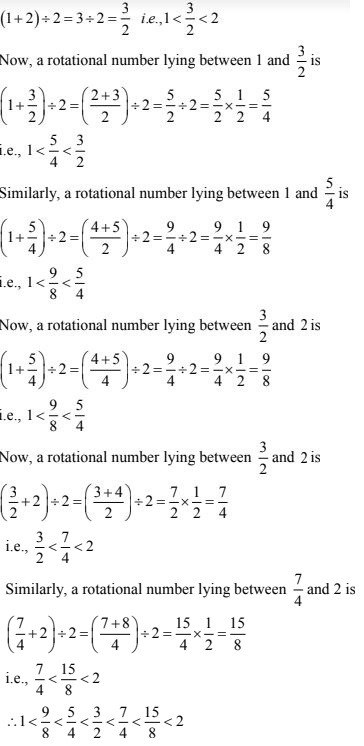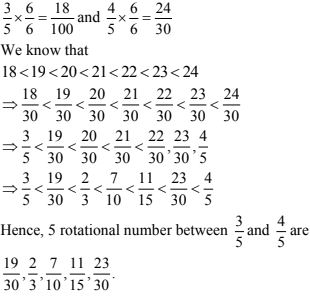# Chapter 1 Number System RD Sharma Solutions Exercise 1.1 Class 9 MathsChapter Name RD Sharma Chapter 1 Number System Exercise 1.1 Book Name RD Sharma Mathematics for Class 10 Other Exercises Exercise 1.2Exercise 1.3Exercise 1.4Exercise 1.5Exercise 1.6 Related Study NCERT Solutions for Class 10 Maths

### Exercise 1.1 Solutions

1. Is zero a rational number ? Can you write it in the form p/q, where p and q are integers and q ≠ 0 ?

Solution

Yes, zero is a rotational number. It can be written in the form of p/q where q to as such as 0/3, 0/5, 0/11, etc...

2. Find five rational numbers between 1 and 2.

Solution

Given to find five rotational numbers between 1 and 2
A rotational number lying between 1 and 2 isRecall that to find a rational number between r and s, you can add r and s and divide the sum by 2, that is (r + s)/2 lies between r and s so, 3/2 is a number between 1 and 2. You can proceed in this manner to find four more rational numbers between 1 and 2, these four numbers are, 5/4, 11/8, 13/8 and 7/4

3. Find six rational numbers between 3 and 4.

Solution

Given to find six rotational number between 3 and 4
We have,4. Find five rational numbers between 3/4 and 4/5

Solution

Given to find 5 rotational numbers lying between 3/5 and 4/5.
We have,5. Are the following statements true or false? Give reasons for your answer.
(i) Every whole number is a rational number.
(ii) Every integer is a rational number.
(iii) Every rational number is a whole number.
(iv) Every natural number is a whole number.
(v) Every integer is a whole number.
(vi) Evert rational number is a whole number.
Solution
(i) False
As whole numbers include zero, whereas natural number does not include zero.

(ii) True
As integers are a part of rotational numbers.

(iii) False
As integers are a part of rotational numbers.

(iv) True
As whole numbers include all the natural numbers.

(v) False
As whole numbers are a part of integers.

(vi) False
As rotational numbers includes all the whole numbers.